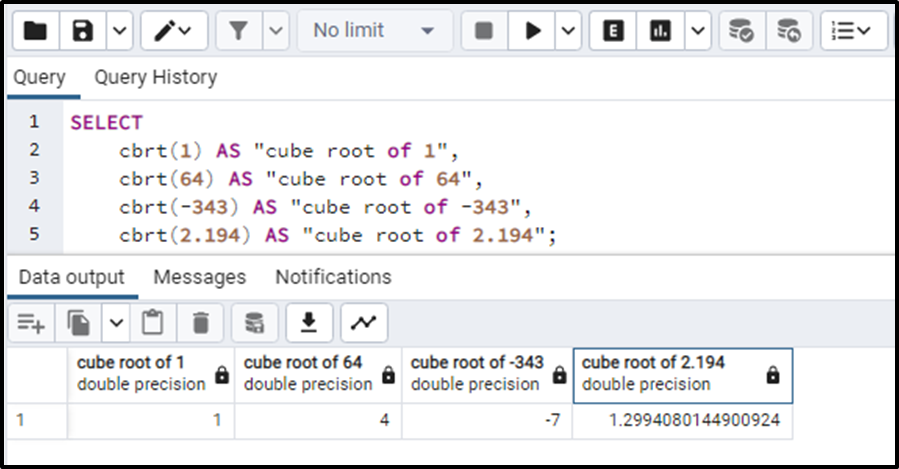##### How to Use cbrt() Function PostgreSQL

The cbrt() function finds the cube root of a number in PostgreSQL. The specified number is passed into the function as an argument. The number is a numeric value that can be positive, negative, zero, decimal, or an integer. The function will return the cube root of the given number.

## How to Use cbrt() Function in PostgreSQL?

The cbrt() function in PostgreSQL takes a numeric value as an input and returns a value having DOUBLE PRECISION data type. The basic syntax for the function is:

`cbrt(Numeric_Value);`

The function returns a NULL if the numeric values passed as an argument are NULL. The cbrt() function will throw an error if the value passed as an argument is of some other data type than numeric.

Let’s see the example of the functioning of this function.

Example

The simple query for the cbrt() function is given below:

```SELECT
cbrt(1) AS "cube root of 1",
cbrt(64) AS "cube root of 64",
cbrt(-343) AS "cube root of -343",
cbrt(2.194) AS "cube root of 2.194";```

The output of the given query will be the additional columns having the values of the cube roots like this:In the above output, we can clearly see that the returned data type of this function is DOUBLE PRECISION. So this was all about cbrt() function.

## Conclusion

The cbrt() function is used to find the cube root of a numeric value. This numeric value needs to be passed into the function. It may contain positive, negative, zero, decimal, or integer values. The cbrt() function gives the cube root of the argument value and that return value will have DOUBLE PRECISION data type.